# How to Perform Arithmetic Across Columns of a MySQL Table Using Python?

• Last Updated : 26 Nov, 2020

Python is a dynamic language, and Python applications can be integrated with database servers. The module used to access a MySQL database from Python is MySQL Connector Python. PyMySQL, MySQLDB and mysqlclient are other Python modules to communicate with a MySQL database server in Python. However, we will use MySQL Connector Python in this article because it is an API written purely in Python which means it does not have dependencies and only requires the standard library.

To perform arithmetic operations on column data, MySQL has Arithmetic Operators. These operators are useful for performing calculations on your data. Arithmetic operators in MySQL are as follows:

In the above table, op1 and op2 can be column names or numeric values (in which case the “from” clause is not required). The following programs will help you understand the use of these operators better.

Database in use:

We will use a product information table in our programs. It consists of the product name, its cost price, selling price, tax and the quantity purchased.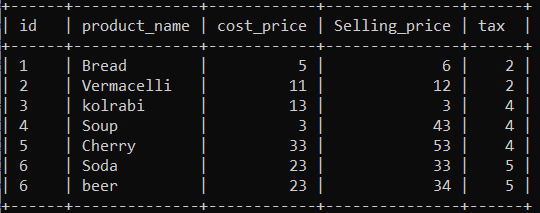Example 1: Use of addition operator

## Python3

 `# Import required packages``import` `mysql.connector`` ` `# Establish connection to MySQL database``mydb ``=` `mysql.connector.connect(``  ``host ``=` `"localhost"``,``  ``user ``=` `"username"``,``  ``password ``=` `"geeksforgeeks"``,``  ``database ``=` `"grocery"``)`` ` `# MySQLCursorDict creates a cursor``# that returns rows as dictionaries``mycursor ``=` `mydb.cursor( dictionary ``=` `True` `)`` ` `# MySQL query for getting total``# sale amount (i.e. selling price + tax)``query ``=` `"SELECT Selling_price, \``                ``tax, \``                ``concat(Selling_price ``+` `tax) AS sale_amount \``         ``FROM product"`` ` `# Execute the query ``mycursor.execute( query )``# Fetch result of query``myresult ``=` `mycursor.fetchall()`` ` `# Print result of query``print``(f``"SP \t Tax \t Sale Amount"``)`` ` `for` `row ``in` `myresult:``   ` `      ``# Each value printed for display purpose (you can simply print row)``    ``print``(f``"{ row[ 'Selling_price' ]} \t { row[ 'tax' ]} \t { row[ 'sale_amount' ]}"``)`` ` `mydb.close()`

Output: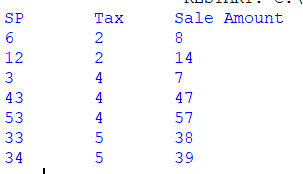Example 2: Use of subtraction operator.

## Python3

 `# Import required packages``import` `mysql.connector`` ` `# Establish connection to MySQL database``mydb ``=` `mysql.connector.connect(``  ``host ``=` `"localhost"` `,``  ``user ``=` `"username"` `,``  ``password ``=` `"geeksforgeeks"` `,``  ``database ``=` `"grocery"``)`` ` `# MySQLCursorDict creates a cursor``# that returns rows as dictionaries``mycursor ``=` `mydb.cursor(dictionary ``=` `True` `)`` ` `# MySQL query for getting profit``# (i.e. selling price - cost price)``query ``=` `"SELECT selling_price, \``                ``cost_price, \``                ``concat(selling_price ``-` `cost_price) AS profit \``         ``FROM product_info"`` ` `# Execute the query ``mycursor.execute( query )`` ` `# Fetch result of query``myresult ``=` `mycursor.fetchall()`` ` `# Print result of query``print``(f``"SP \t CP \t Profit"``)``for` `row ``in` `myresult:``  ``print``(f``"{ row[ 'selling_price' ]} \t { row[ 'cost_price' ]} \t { row[ 'profit' ]}"``)`` ` `mydb.close()`

Output: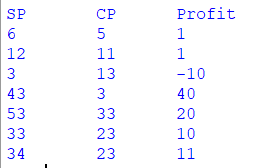Example 3: Use of multiplication operator

## Python3

 `# Import required packages``import` `mysql.connector`` ` `# Establish connection to MySQL database``mydb ``=` `mysql.connector.connect(``  ``host ``=` `"localhost"` `,``  ``user ``=` `"username"` `,``  ``password ``=` `"geeksforgeeks"` `,``  ``database ``=` `"grocery"``)`` ` `# MySQLCursorDict creates a cursor that returns rows as dictionaries``mycursor ``=` `mydb.cursor(dictionary ``=` `True``)`` ` `# MySQL query for getting total amount (i.e. sale amount * quantity)``query ``=` `"SELECT selling_price, \``                ``tax, \``                ``concat((selling_price ``*` `tax)) AS total_amount \``         ``FROM product_info"`` ` `# Execute the query ``mycursor.execute( query )`` ` `# Fetch result of query``myresult ``=` `mycursor.fetchall()`` ` `# Print result of query``print``(f``"SP \t Tax \t Total"``)``for` `row ``in` `myresult:``  ``print``(f``"{row[ 'selling_price' ]} \t { row[ 'tax' ]}\t{row['total_amount']}"``)`` ` `# Close database connection``mydb.close()`

Output: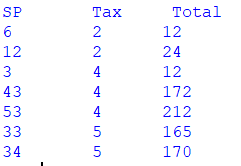Example 4: Use of division operator.

## Python3

 `# Import required packages``import` `mysql.connector`` ` `# Establish connection to MySQL database``mydb ``=` `mysql.connector.connect(``  ``host ``=` `"localhost"` `,``  ``user ``=` `"username"` `,``  ``password ``=` `"geeksforgeeks"` `,``  ``database ``=` `"grocery"``)`` ` `# MySQLCursorDict creates a cursor``# that returns rows as dictionaries``mycursor ``=` `mydb.cursor( dictionary ``=` `True` `)`` ` `# MySQL query for getting halved selling price of all products``query ``=` `"SELECT selling_price, \``                ``concat(selling_price ``/` `2``) AS discount_price \``         ``FROM product_info"`` ` `# Execute the query ``mycursor.execute( query )`` ` `# Fetch result of query``myresult ``=` `mycursor.fetchall()`` ` `# Print result of query``print``(f``"SP \t Discounted Price"``)``for` `row ``in` `myresult:``  ``print``(f``"{ row[ 'selling_price' ]} \t { row ['discount_price']}"``)`` ` `# Close database connection``mydb.close()`

Output: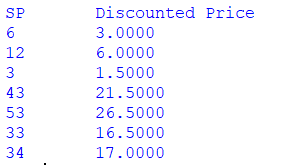Example 5: Use of modulus operator.

## Python3

 `# Import required packages``import` `mysql.connector`` ` `# Establish connection to MySQL database``mydb ``=` `mysql.connector.connect(``  ``host ``=` `"localhost"` `,``  ``user ``=` `"username"` `,``  ``password ``=` `"geeksforgeeks"` `,``  ``database ``=` `"grocery"``)`` ` `# MySQLCursorDict creates a cursor``# that returns rows as dictionaries``mycursor ``=` `mydb.cursor(dictionary ``=` `True``)`` ` `# MySQL query for getting remainder``query ``=` `"SELECT selling_price, \``                ``cost_price, \``                ``concat(selling_price ``%` `cost_price) AS mod_example \``         ``FROM product_info"`` ` `# Execute the query ``mycursor.execute( query )`` ` `# Fetch result of query``myresult ``=` `mycursor.fetchall()`` ` `# Print result of query``print``(f``"SP \t Qnty \t MOD"``)``for` `row ``in` `myresult:``  ``print``(f"{ row [ ``'selling_price'` `]}``              ``\t { row [ ``'cost_price'` `]}``                  ``\t{row[ ``'mod_example'` `]}")`` ` `# Close database connection``mydb.close()`

Output: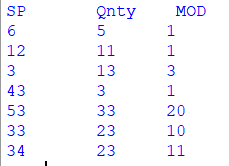My Personal Notes arrow_drop_up